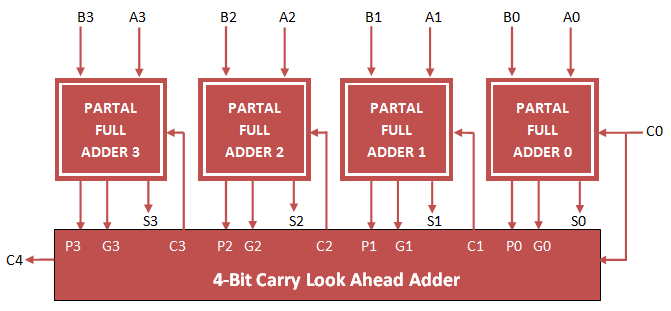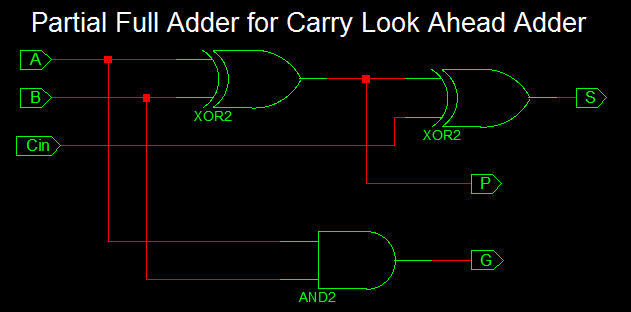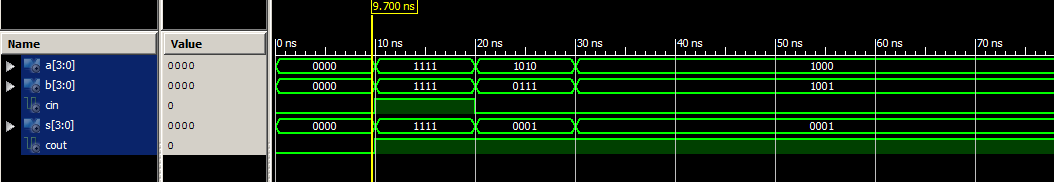# Carry Look Ahead Adder VHDL Code

Carry Look Ahead Adder is fastest adder compared to Ripple carry Adder. For the Purpose of carry Propagation, Carry look Ahead Adder construct Partial Full Adder, Propagation and generation Carry block. It avoid Carry propagation through each adder.

In order to implement Carry Look Ahead Adder, first implement Partial Full Adder and then Carry logic using Propagation and generation Block.Partial Full Adder consist of inputs (A, B, Cin) and Outputs (S, P, G) where P is Propagate Output and G is Generate output.

VHDL code for carry look ahead adder can be implemented by first constructing Partial full adder block and port map them to four times and also implementing carry generation block as shown below.### VHDL Code for Partial Full Adder

```
library IEEE;
use IEEE.STD_LOGIC_1164.ALL;

Port ( A : in STD_LOGIC;
B : in STD_LOGIC;
Cin : in STD_LOGIC;
S : out STD_LOGIC;
P : out STD_LOGIC;
G : out STD_LOGIC);

architecture Behavioral of Partial_Full_Adder is

begin

S <= A xor B xor Cin;
P <= A xor B;
G <= A and B;

end Behavioral;

```

### VHDL Code for Carry Look Ahead Adder

```
library IEEE;
use IEEE.STD_LOGIC_1164.ALL;

Port ( A : in STD_LOGIC_VECTOR (3 downto 0);
B : in STD_LOGIC_VECTOR (3 downto 0);
Cin : in STD_LOGIC;
S : out STD_LOGIC_VECTOR (3 downto 0);
Cout : out STD_LOGIC);

architecture Behavioral of Carry_Look_Ahead is

Port ( A : in STD_LOGIC;
B : in STD_LOGIC;
Cin : in STD_LOGIC;
S : out STD_LOGIC;
P : out STD_LOGIC;
G : out STD_LOGIC);
end component;

signal c1,c2,c3: STD_LOGIC;
signal P,G: STD_LOGIC_VECTOR(3 downto 0);
begin

PFA1: Partial_Full_Adder port map( A(0), B(0), Cin, S(0), P(0), G(0));
PFA2: Partial_Full_Adder port map( A(1), B(1), c1, S(1), P(1), G(1));
PFA3: Partial_Full_Adder port map( A(2), B(2), c2, S(2), P(2), G(2));
PFA4: Partial_Full_Adder port map( A(3), B(3), c3, S(3), P(3), G(3));

c1 <= G(0) OR (P(0) AND Cin);
c2 <= G(1) OR (P(1) AND G(0)) OR (P(1) AND P(0) AND Cin);
c3 <= G(2) OR (P(2) AND G(1)) OR (P(2) AND P(1) AND G(0)) OR (P(2) AND P(1) AND P(0) AND Cin);
Cout <= G(3) OR (P(3) AND G(2)) OR (P(3) AND P(2) AND G(1)) OR (P(3) AND P(2) AND P(1) AND G(0)) OR (P(3) AND P(2) AND P(1) AND P(0) AND Cin);

end Behavioral;

```

### VHDL Testbench Code for Carry Look Ahead Adder

```
LIBRARY ieee;
USE ieee.std_logic_1164.ALL;

ARCHITECTURE behavior OF Tb_Carry_Look_Ahead IS

-- Component Declaration for the Unit Under Test (UUT)

PORT(
A : IN std_logic_vector(3 downto 0);
B : IN std_logic_vector(3 downto 0);
Cin : IN std_logic;
S : OUT std_logic_vector(3 downto 0);
Cout : OUT std_logic
);
END COMPONENT;

--Inputs
signal A : std_logic_vector(3 downto 0) := (others => '0');
signal B : std_logic_vector(3 downto 0) := (others => '0');
signal Cin : std_logic := '0';

--Outputs
signal S : std_logic_vector(3 downto 0);
signal Cout : std_logic;

BEGIN

-- Instantiate the Unit Under Test (UUT)
uut: Carry_Look_Ahead PORT MAP (
A => A,
B => B,
Cin => Cin,
S => S,
Cout => Cout
);

-- Stimulus process
stim_proc: process
begin
-- hold reset state for 100 ns.
wait for 10 ns;

A <= "1111";
B <= "1111";
Cin <= '1';

wait for 10 ns;

A <= "1010";
B <= "0111";
Cin <= '0';

wait for 10 ns;

A <= "1000";
B <= "1001";
Cin <= '0';

wait;

end process;

END;

```

### Output Waveform for Carry Look Ahead Adder VHDL Code### 2 thoughts on “Carry Look Ahead Adder VHDL Code”

1.excellent

2.Excellent